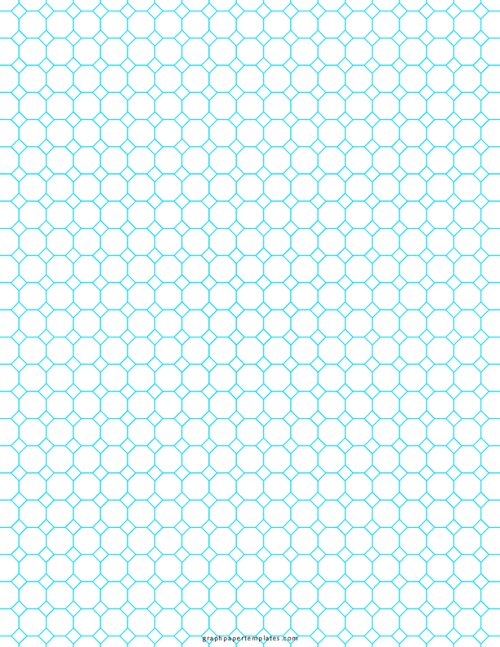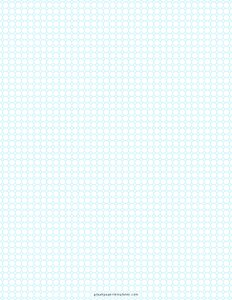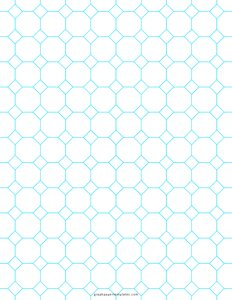# 1/2" inch Octagon Graph PaperPaper Size: Letter

Format: PDF

Size: 8.5" x 11"  -  ( 216 mm x 279 mm )

Layout: Portrait / Vertical

Color: Blue

Octagon graph paper with half inch spacing is commonly used for various purposes, including graphing equations, plotting polygons, and creating tessellations.

## Printable 1/2" Inch Octagon Graph Paper

This type of graph paper can be particularly useful for visualizing complex mathematical concepts. Octagon graph paper is also often used by architects and designers to create three-dimensional renderings of buildings and other structures.## Quarter Inch (1/4")

Quarter Inch Octagon graph paper can be useful for various purposes, from creating tessellations to drawing three-dimensional objects.## One Inch

Octagon Graph Paper Printable PDF with 1" inch spacing. Perfect for various mathematical and engineering applications. It is most commonly used for enlargement designs in mathematical and engineering projects.

In addition, octagon graph paper can be used for various crafts projects, such as making origami or quilting. Whether you are a student, a professional, or a hobbyist, this versatile tool can be a valuable asset in your design arsenal.

1/2" inch Octagon Graph Paper

### You may also like:

Engineering Graph Paper

Engineering graph paper is a type of graph paper with horizontal and vertical lines at equal distance with major lines appearing at a specified distance of 1/2 inch, 1/4th, 1/5th, 1/10th, or every 5 or 10 lines.

10 Squares Per Inch Graph Paper

10 squares per inch graph paper PDF printable has 10 squares per inch. It is perfect for graphing and drawing small diagrams. It can be used for a variety of purposes, from sketching and drawing to math and science.